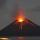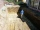# Area of shape + volume - math problems

#### Number of problems found: 138

• Pyramid heightFind the volume of a regular triangular pyramid with edge length a = 12cm and pyramid height h = 20cm.
• Tetrahedral pyramidCalculate the surface S and the volume V of a regular tetrahedral pyramid with the base side a = 5 m and a body height of 14 m.
• ContainerThe container has a cylindrical shape the base diameter 0.8 m and the area of the base is equal to the area of the wall. How many liters of water can we pour into the container?In a regular quadrilateral pyramid, the height is 6.5 cm and the angle between the base and the side wall is 42°. Calculate the surface area and volume of the body. Round calculations to 1 decimal place.
• SandpileAuto sprinkled with sand to an approximately conical shape. Workers wanted to determine the volume (amount of sand) and therefore measure the base's circumference and the length of both sides of the cone (over the top). What is the sand cone's volume if t
• VolcanoThe crater of a volcano is approximately in the shape of a cone of a base 3.1416 sq. Mi. The crater's depth is 1500 ft. How many cubic yards of earth would be required to fill this cavity?
• 3s prismIt is given a regular perpendicular triangular prism with a height 19.0 cm and a base edge length 7.1 cm. Calculate the volume of the prism.
• Square prismCalculate the volume of a square prism of high 2 dm wherein the base is: rectangle with sides 17 cm and 1.3 dm
• Rectangle 35Find the area of a rectangle when the diagonal is equal to 30 cms and the width is double the length.
• The EarthThe Earth's surface is 510,000,000 km2. Calculates the radius, equator length, and volume of the Earth, assuming the Earth has the shape of a sphere.
• The trenchCalculate how many cubic meters of soil needs to be removed from the excavation in the shape of an isosceles trapezoid, the top width is 3 meters, the lower width is 1.8 m, the depth of the excavation is 1 m, and the length is 20 m.
• Hexa prismDetermine the volume of hex prism with edge base 4 cm. The body height is 28 cm.
• Base of houseCalculate the volume of the bases of a square house, if the base depth is 1.2 m, the width is 40 cm and their outer circumference is 40.7 m.A regular quadrilateral pyramid has a volume of 24 dm3 and a base edge a = 4 dm. Calculate: a/height of the pyramid b/sidewall height c/surface of the pyramid
• Triangular pyramidCalculate the volume of a regular triangular pyramid with edge length a = 12cm and pyramid height v = 20cm.
• Surface of the coneCalculate the surface of the cone if its height is 8 cm and the volume is 301.44 cm3.
• Octagonal pyramidFind the volume of a regular octagonal pyramid with height v = 100 and the angle of the side edge with the plane of the base is α = 60°.
• Truncated pyramidHow many cubic meters is the volume of a regular four-sided truncated pyramid with edges one meter and 60 cm and high 250 mm?
• Triangular prism,The regular triangular prism, whose edges are identical, has a surface of 2514 cm ^ 2 (square). Find the volume of this body in cm3 (l).
• Juice boxThe juice box has a volume of 200ml with its base is an isosceles triangle with sides a = 4,5cm and a height of 3,4cm. How tall is the box?

Do you have an interesting mathematical word problem that you can't solve it? Submit a math problem, and we can try to solve it.

We will send a solution to your e-mail address. Solved examples are also published here. Please enter the e-mail correctly and check whether you don't have a full mailbox.

Please do not submit problems from current active competitions such as Mathematical Olympiad, correspondence seminars etc...

Tip: Our volume units converter will help you with the conversion of volume units. Examples of area of plane shapes. Volume - math word problems.